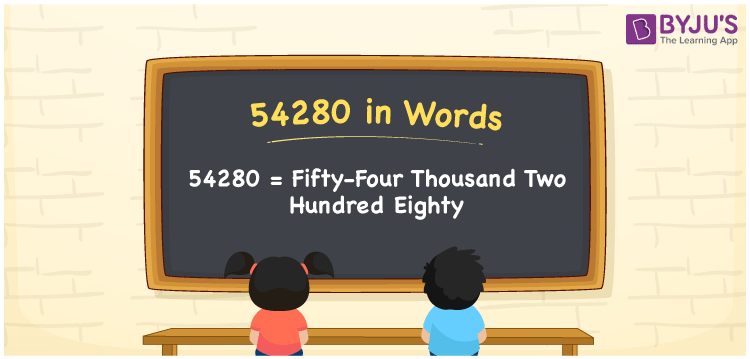# 54280 in Words

We can write 54280 in words as Fifty-four thousand two hundred eighty. If you paid an annual tariff of Rs. 54280, you may communicate it as ” I paid Fifty-four thousand two hundred eighty rupees as an annual tariff”. Also, 54280 is the cardinal number since it denotes a specific quantity or amount.

 54280 in words Fifty-four thousand two hundred eighty Fifty-four thousand two hundred eighty in Numbers 54280

## 54280 in English words

We generally write numbers in words using the English alphabet. Thus, we can spell 54280 in English as “Fifty-four thousand two hundred eighty”.## How to Write 54280 in Words?

In this section, you will learn how to convert the number 54280 to word form using a five-column place value chart since it contains five digits. This can be done as follows.

 Ten thousands Thousands Hundreds Tens Ones 5 4 2 8 0

Here, ones = 0, tens = 8, hundreds = 2, thousands = 4, ten thousands = 5.

These numbers can be expanded as:

5 × Ten thousand + 4 × Thousand + 2 × Hundred + 8 × Ten + 0 × One

= 5 × 10000 + 4 × 1000 + 2 × 100 + 8 × 10 + 0 × 1

= 50000 + 4000 + 200 + 80

= Fifty thousand + Four thousand + Two hundred + Eighty

= Fifty-four thousand two hundred eighty

Therefore, 54280 in words = Fifty-four thousand two hundred eighty.

### Facts About the Number 54280

54280 is a natural number that is the successor of 54279 and the predecessor of 54281.

54280 in words – Fifty-four thousand two hundred eighty

Is 54280 an odd number? – No

Is 54280 an even number? – Yes

Is 54280 a prime number? – No

Is 54280 a composite number? – Yes

Is 54280 a perfect square number? – No

Is 54280 a perfect cube number? – No

## Frequently Asked Questions on 54280 in Words

Q1

### How do you write 54280 in English words?

In English words, we can write the number 54280 as Fifty-four thousand two hundred eighty.
Q2

### How to write Rs. 54280 in words on a cheque?

On a cheque, we generally write an amount of Rs. 54280 in words as “Fifty-four thousand two hundred eighty rupees only”.
Q3

### What is the number name for 54280?

The number name for 54280 is Fifty-four thousand two hundred eighty.